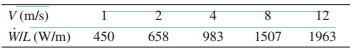### Create an Account

Home / Questions / A series of experiments were conducted by passing 40C air over a long 25 mm diameter cylin...

# A series of experiments were conducted by passing 40C air over a long 25 mm diameter cylinder with an embedded electrical heater The objective of these experiments was to determine the power per

A series of experiments were conducted by passing 40°C air over a long 25 mm diameter cylinder with an embedded electrical heater. The objective of these experiments was to determine the power per unit length required (W/L) to maintain the surface temperature of the cylinder at 300ºC for different air velocities (V). The results of these experiments are given in the following table:(a) Assuming a uniform temperature over the cylinder, negligible radiation between the cylinder surface and surroundings, and steady state conditions, determine the convection heat transfer coefficient (h) for each velocity (V). Plot the results in terms of h (W/m2 ·K) vs. V (m/s). Provide a computer generated graph for the display of your results and tabulate the data used for the graph.

(b) Assume that the heat transfer coefficient and velocity can be expressed in the form of h = CVn. Determine the values of the constants C and n from the results of part (a) by plotting h vs. V on log-log coordinates and choosing a C value that assures a match at V = 1 m/s and then varying n to get the best fit.

Jul 22 2020 View more View LessSubscribe To Get Solution